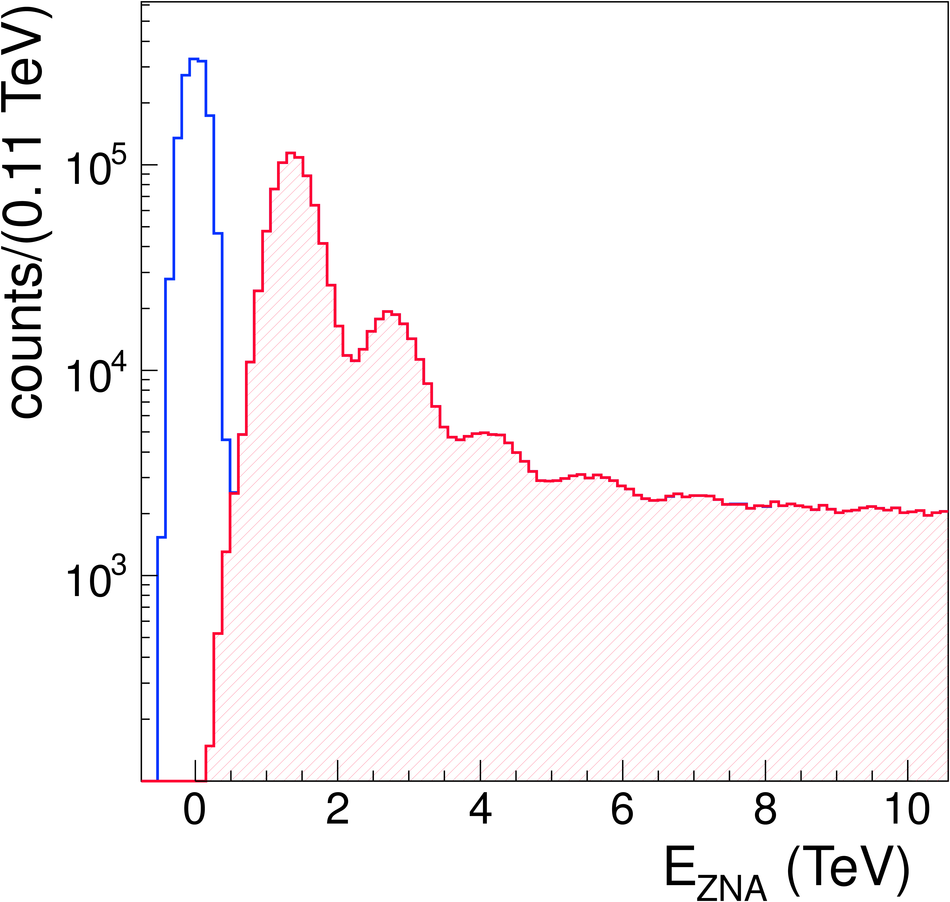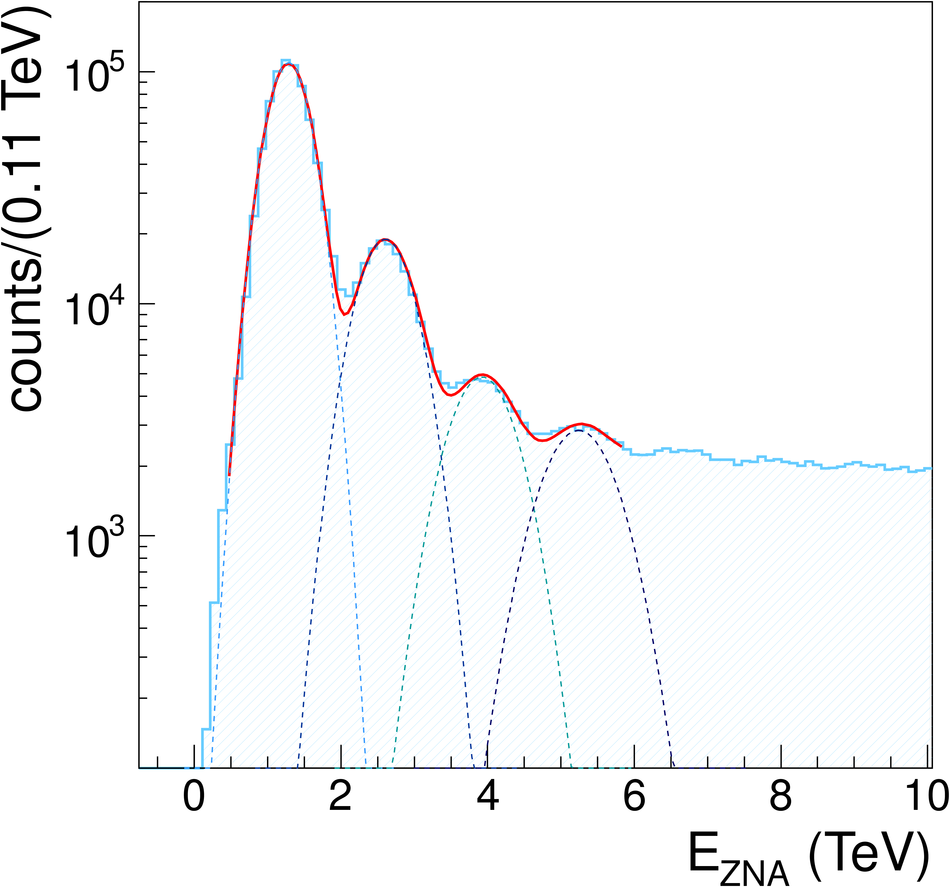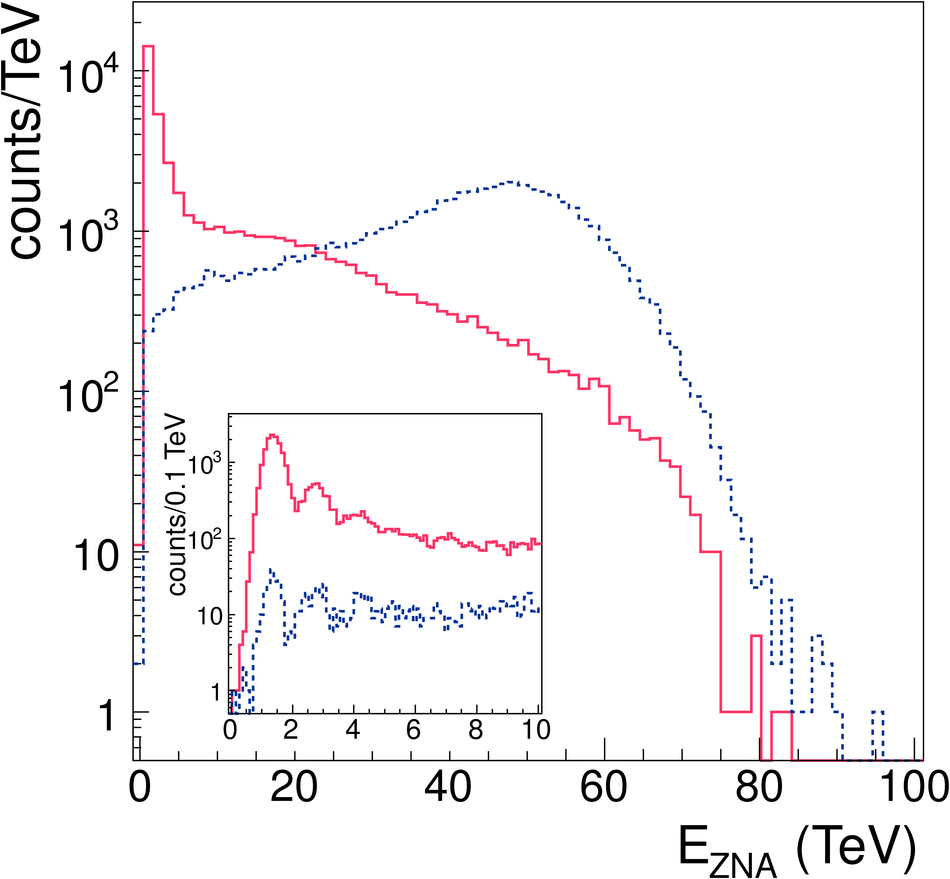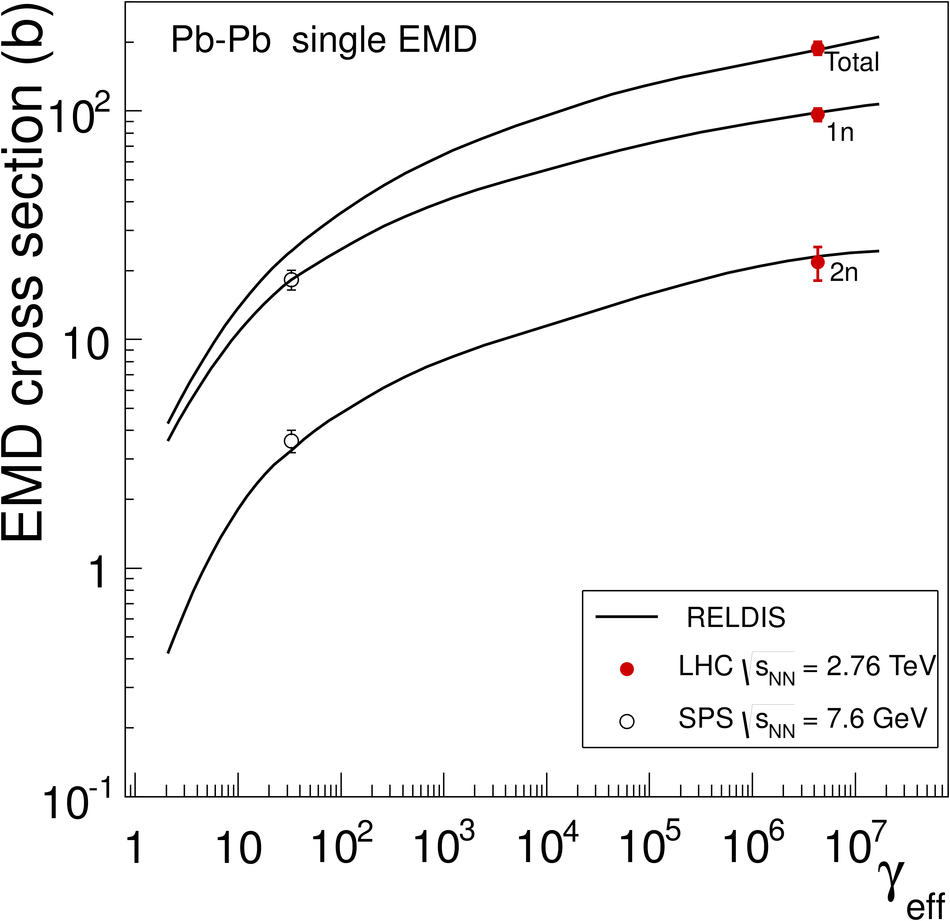# Measurement of the cross section for electromagnetic dissociation with neutron emission in Pb-Pb collisions at $\sqrt{s_{\rm NN}}=2.76$ TeV

The first measurement of neutron emission in electromagnetic dissociation of $^{208}$Pb nuclei at the LHC is presented. The measurement is performed using the neutron Zero Degree Calorimeters of the ALICE experiment, which detect neutral particles close to beam rapidity. The measured cross sections of single and mutual electromagnetic dissociation of Pb nuclei at $\sqrt{s_{\rm NN}}$ = 2.76 TeV with neutron emission are $\sigma_{\rm single\ EMD} = 187.4\pm0.2$ (stat.) $^{+13.2} _{-11.2}$ (syst.) b and $\sigma_{\rm mutual\ EMD} = 5.7\pm0.1$ (stat.) $\pm$0.4 (syst.) b, respectively. The experimental results are compared to the predictions from a relativistic electromagnetic dissociation model.

Figures

## Figure 1

 Energy deposition in ZNC versus ZNA for single EMD plus hadronic events. The 1n signal is at 1.38 TeV. The events where at least 1n is detected by both ZNs are associated to mutual EMD and hadronic processes.## Figure 2

 ZNA energy spectrum requiring signal over threshold in ZNA or ZNC (not filled area) superimposed to ZNA energy spectrum requiring signal in ZNA (shaded area). The first peak centered at $E=0$ corresponds to pedestal events,where no signal from neutron emission is detected by the ZNA.## Figure 3

 ZNA energy spectrum requiring signal over threshold in ZNA but not in ZNC, rejecting thus neutron emission on the opposite side. The dashed lines represent the single fits of the different peaks (1n, 2n,...), while the continuous line is the sum of all the contributions.## Figure 4

 ZNA energy spectrum for mutual EMD (no signal in any ZEM, continuous line) and hadronic (a signal in at least one of the two ZEMs, dashed line) event selection. The insert shows an expanded view of the low energy region.## Figure 5

 Total single EMD cross sections and partial EMD cross sections foremission of one and two neutrons as a function of the effective Lorentz factor $\gamma_{\rm eff}$ The closed symbols are our data, while the open symbols represent the results obtained at CERN SPS at 30 GeV The RELDIS predictions for total, 1n and 2n EMD cross sections are shown as solid lines.